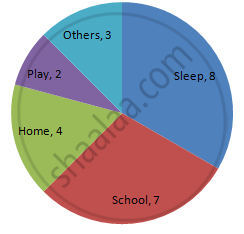Advertisement Remove all ads

# The Number of Hours, Spent by a School Boy on Different Activities in a Working Day, is Given Below: - Mathematics

Sum

The number of hours, spent by a school boy on different activities in a working day, is given below:

 Activities Sleep School Home Play Others Total Number of hours 8 7 4 2 3 24

Present the information in the form of a pie-chart.

Advertisement Remove all ads

#### Solution

We know:
Central angle of a component = (component value / sum of component values × 360)
Here, total number of hours = 24
Thus, the central angle for each component can be calculated as follows:

 Activity Number of hours Sector angle Sleep 8 8/24 ×× 360 = 120o School 7 7/24 ×× 360 = 105o Home 4 4/24 ×× 360 = 60o Play 2 2/24 ×× 360 = 30o Others 3 3/24 ×× 360 = 45o

Now, the pie chat that represents the given data can be constructed by following the steps given below:
Step 1 : Draw a circle of an appropriate radius.
Step 2 : Draw a vertical radius of the circle drawn in step 1.
Step 3 : Choose the largest central angle. Here, the largest central angle is 120o. Draw a sector with the central angle 120o in such a way that one of its radii coincides with the radius drawn in step 2 and another radius is in its counter-clockwise direction.
Step 4 : Construct other sectors representing other items in the clockwise direction in descending order of magnitudes of their central angles.
​Step 5 : Shade the sectors with different colours and label them as shown as in the figure below.Is there an error in this question or solution?
Advertisement Remove all ads

#### APPEARS IN

RD Sharma Class 8 Maths
Chapter 25 Data Handling-III (Pictorial Representation of Data as Pie Charts or Circle Graphs)
Exercise 25.1 | Q 1 | Page 12
Advertisement Remove all ads

#### Video TutorialsVIEW ALL 

Advertisement Remove all ads
Share
Notifications

View all notifications

Forgot password?